### Home | Up One Level | Previous | Next

WALTER RITZ

#### Annales 237 (Oeuvres 394)

We observe this action in the study of cathode rays and canal rays, where the speed v of the ion e in motion, while remaining very much inferior to that of light, is very large in relation to the speed of the ions of the closed current, then in Hall’s phenomena, the Zeeman effect and the magnetic rotation of the polarization plane of light.

Let us keep the notations of the preceding section; the action undergone by e will be the sum of the actions of the positive and negative chargesof all the elements of volume of the current. The conductor itself, in these experiments, is at rest; thus V'=0,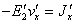and the speed v' of the negative ions, the only ones mobile by hypothesis, is small in relation to v. The general formula (13) gives for a linear element ds' of the closed current, and the abstraction made from the electrostatic term and

the accelerations,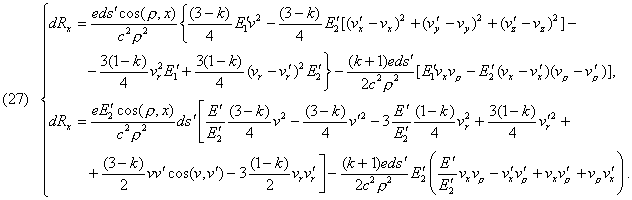(Oeuvres 395) Beside the terms proportional to v, v', the terms in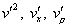disappear becauseis very small; it is the same for the terms infor they relate to the electrostatic action of the charges E' of the current as, that is they are very small. There remain the terms proportional to v and v' which may be written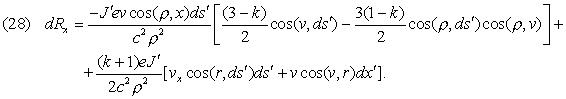This is formula (25) in a different notation. The current ds' being closed, we may, as we have done previously, integrate in relation to s', and we will again obtain

the result that the action of a magnet or a closed current at rest, on an ion in movement is the same as in Lorentz’s theory.

If v becomes smaller and comparable to v', all the terms of (27) become very small, and the phenomenon necessitates, in order to be observed, very delicate means of investigation. This is what takes place when in placing a plate carrying a current between the poles of an electromagnet, one observes, when the magnet is energized, a difference of potential between the two sides of the current (the Hall effect). The electrostatic action of the magnet being negligible, it is a fortiori the same for terms in E' of formula (27); thus there will be, firstly, an action proportional to J' and v', which is the same, as we have seen, in both theories; there is, moreover, the forceThe latter depends, as does Hall’s phenomenon itself (and contrary to the forces studied until now) on the hypotheses made on the movement of ions: it would be sensibly nil if the positive and negative ions of the electromagnet had equal speeds (Oeuvres 396) and opposite signs. Formula (20) of Lorentz gives analogous forces: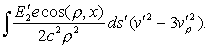The term in v'2 has the nature of an electric force performed by the elements of volume of the magnet independently of their orientation (the molecular currents being considered as permanent and simply oriented by the magnetization). These actions, independent of the sign of the magnetic field and of the existence of a current in

the plate, will no doubt escape even the most delicate observations. As to the terms containing the accelerations of the ions of the current, and which are the same in both theories, they are equally negligible for analogous motives.

  Lorentz, Elektronentheorie, p. 217.